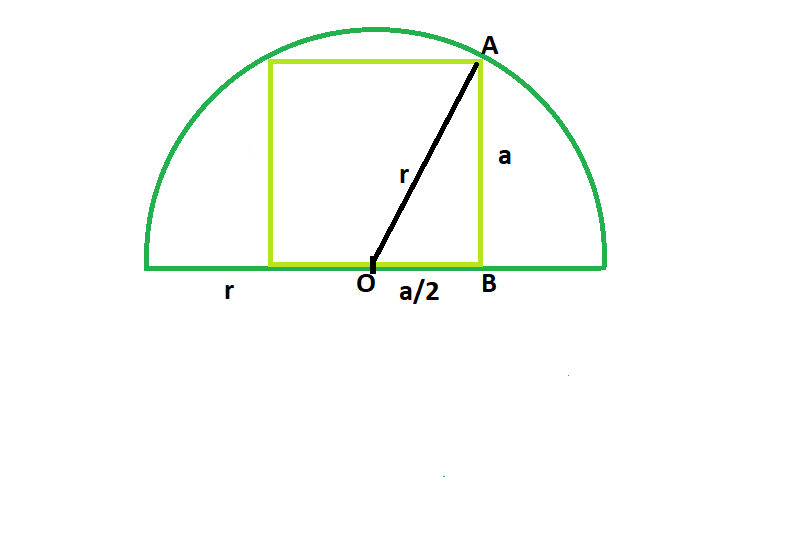# Largest square that can be inscribed in a semicircle

Given a semicircle with radius r, we have to find the largest square that can be inscribed in the semicircle, with base lying on the diameter.

Examples:

```Input: r = 5
Output: 20

Input: r = 8
Output: 51.2
```

## Recommended: Please try your approach on {IDE} first, before moving on to the solution.Approach: Let r be the radius of the semicircle & a be the side length of the square.
From the figure we can see that, centre of the circle is also the midpoint of the base of the square.So in the right angled triangle AOB, from Pythagorus Theorem:

a^2 + (a/2)^2 = r^2

5*(a^2/4) = r^2

a^2 = 4*(r^2/5) i.e. area of the square

Below is the implementation of the above approach:

## C++

 `// C++ Program to find the biggest square ` `// which can be inscribed within the semicircle ` `#include ` `using` `namespace` `std; ` ` `  `// Function to find the area ` `// of the squaare ` `float` `squarearea(``float` `r) ` `{ ` ` `  `    ``// the radius cannot be negative ` `    ``if` `(r < 0) ` `        ``return` `-1; ` ` `  `    ``// area of the square ` `    ``float` `a = 4 * (``pow``(r, 2) / 5); ` ` `  `    ``return` `a; ` `} ` ` `  `// Driver code ` `int` `main() ` `{ ` `    ``float` `r = 5; ` `    ``cout << squarearea(r) << endl; ` ` `  `    ``return` `0; ` `} `

## Java

 `// Java Program to find the biggest square ` `// which can be inscribed within the semicircle ` ` `  `import` `java.io.*; ` ` `  `class` `GFG { ` ` `  ` `  `// Function to find the area ` `// of the squaare ` `static` `float` `squarearea(``float` `r) ` `{ ` ` `  `    ``// the radius cannot be negative ` `    ``if` `(r < ``0``) ` `        ``return` `-``1``; ` ` `  `    ``// area of the square ` `    ``float` `a = ``4` `* (``float``)(Math.pow(r, ``2``) / ``5``); ` ` `  `    ``return` `a; ` `} ` ` `  `// Driver code ` ` `  `    ``public` `static` `void` `main (String[] args) { ` `         ``float` `r = ``5``; ` `    ``System.out.println( squarearea(r)); ` `    ``} ` `} ` `// This code is contributed by chandan_jnu. `

## Python3

 `# Python 3 program to find the  ` `# biggest square which can be  ` `# inscribed within the semicircle ` ` `  `# Function to find the area ` `# of the squaare ` `def` `squarearea(r): ` ` `  `    ``# the radius cannot be ` `    ``# negative ` `    ``if` `(r < ``0``): ` `        ``return` `-``1` ` `  `    ``# area of the square ` `    ``a ``=` `4` `*` `(``pow``(r, ``2``) ``/` `5``) ` ` `  `    ``return` `a ` ` `  `# Driver code ` `if` `__name__ ``=``=` `"__main__"``: ` `     `  `    ``r ``=` `5` `    ``print``(``int``(squarearea(r))) ` ` `  `# This code is contributed ` `# by ChitraNayal `

## C#

 `// C# Program to find the  ` `// biggest square which can be ` `// inscribed within the semicircle ` `using` `System; ` ` `  `class` `GFG ` `{ ` ` `  `// Function to find the  ` `// area of the squaare ` `static` `float` `squarearea(``float` `r) ` `{ ` ` `  `    ``// the radius cannot be negative ` `    ``if` `(r < 0) ` `        ``return` `-1; ` ` `  `    ``// area of the square ` `    ``float` `a = 4 * (``float``)(Math.Pow(r, 2) / 5); ` ` `  `    ``return` `a; ` `} ` ` `  `// Driver code ` `public` `static` `void` `Main () ` `{ ` `    ``float` `r = 5; ` `    ``Console.WriteLine(squarearea(r)); ` `} ` `} ` ` `  `// This code is contributed  ` `// by anuj_67 `

## PHP

 ` `

Output:

```20
```

Attention reader! Don’t stop learning now. Get hold of all the important DSA concepts with the DSA Self Paced Course at a student-friendly price and become industry ready.

My Personal Notes arrow_drop_upBudding Web DeveloperKeen learnerAverage CoderDancer&Social Activist

If you like GeeksforGeeks and would like to contribute, you can also write an article using contribute.geeksforgeeks.org or mail your article to contribute@geeksforgeeks.org. See your article appearing on the GeeksforGeeks main page and help other Geeks.

Please Improve this article if you find anything incorrect by clicking on the "Improve Article" button below.

Article Tags :
Practice Tags :

Be the First to upvote.

Please write to us at contribute@geeksforgeeks.org to report any issue with the above content.